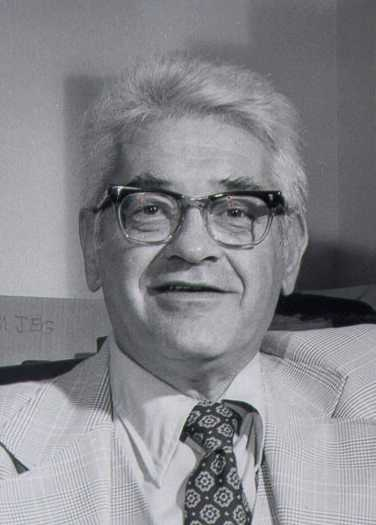Enter the content which will be displayed in sticky bar

# MemberDr. Petr Beckmann
local time: 2020-12-01 01:49 (-06:00 DST)
Dr. Petr Beckmann (Abstracts)
Titles
Abstracts Details
• Sagnac and Gravitation (1992) [Updated 9 years ago]

The Einstein theory is sometimes incorrectly accused of being unable to explain the Sagnac effect (even for an unclosed path configuration). However, its explanation reveals that it works with the gravitational field as the equivalent of an ether, in which the velocity of light is not constant unless space is distorted into a non-Euclidean geometry so as to force its constancy. Although not wrong in the sense of disagreeing with experimental results, this mathematical formalism is complicated, opaque and unnecessary, for it is easily replaced by very simple mathematics in Euclidean space yielding the same result when it is assumed that the velocity of light is constant with respect to the local gravitational field; this requires no special non-Euclidean space.

• A Seductive Fallacy (1991) [Updated 9 years ago]

It has been claimed since at least 1910 that the Lorentz Transformation and Einstein's Theory of Relativity are derivable without using the constancy of the velocity of light as a postulate. The proof makes three (in some versions, more) very basic and natural assumptions, and derives the Lorentz transformation with a constant velocity of light as the only possible transformation between moving inertial frames that satisfies experimental observations. It is shown that the proof is flawed by illegitimately interpreting a constant that has the dimensions of a velocity as the velocity of light, and by claiming that this is the only choice confirmed by experiment.

• The Double-Slit Paradox (1990) [Updated 9 years ago]

Summary: It is shown directly from the Maxwell equations that the velocity of an electron with average v will oscillate about that value, giving rise to a non-radiating electromagnetic wave in the neighborhood of the electron. The oscillations are characterized by the de Broglie relation, which is normally simply postulated. Applying the result, the wave-particle paradox of electron diffraction by a double slit is resolved: the electron goes through only one aperture, but the wave surrounding it is diffracted by both of them. In the neighborhood of the electron the group velocity of the diffracted field is slowed to that of the electron, giving rise to the observed diffraction pattern.

• [Updated 9 years ago]

The optical part of the trajectory of light in a gravitational field is derived from Hayden's expression for the refractive index and Fermat's principle. The bending angle obtainable from it agrees with Hayden's result. Unlike the approximate trajectory derived under the assumptions of Einstein's General Theory, the trajectory derived from Fermat's principle is an exact result in closed form.

• Electron Clusters (1990) [Updated 9 years ago]

A Texas research company has consistently produced electron clusters of some 1010 electrons within the space of a few microns, traveling along the boundary of a dielectric at about 0.1 of the velocity of light. The paper gives a possible explanation of the phenomenon, which at first sight seems to contradict Coulomb's Law.  It is based on the force of the oscillation Faraday field surrounding a moving electron as previously derived by the author.